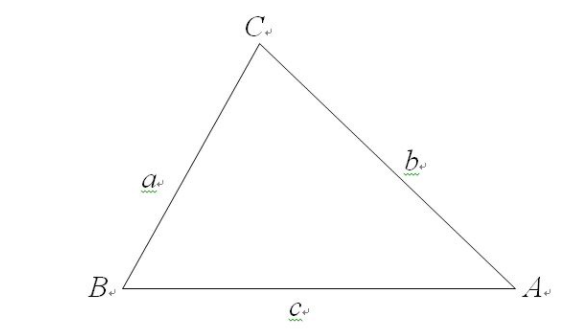## python3类怎么学?python数学应用

240次阅读```def ...:    # 参照公式把五个函数定义出来，就不详细写了
...
def ...:
...

# 然后调用定义好的函数，传入边长数据
angleA(6,7,8)  # 计算角A
->0.8127555613686607  # 注意返回值为弧度

angleB(6,7,8)  # 计算角B
->1.0107210205683146

angleC(6,7,8)  # 计算角C
->1.318116071652818

square(6,7,8)  # 计算面积
->20.33316256758894

circle(6,7,7)  # 计算周长，额，好像有个数字写错了
->20  # 计算结果当然也就错了```

```def calculate(a,b,c):
angleA = ...
angleB = ...
angleC = ...
square = ...
circle = ...
return {'角A':angleA, '角B':angleB, '角C':angleC, '面积':square, '周长':circle}

result=calculate(6,7,8)

result['角A']
->0.8127555613686607

result['面积']
->20.33316256758894```